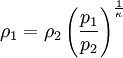A adiabatic (also: adiabate; Greek α [a] - not, διαβαίνειν [diabaínein] - go through) Change of state is a thermodynamic process in which a system is transferred from one state to another without exchanging heat energy with its surroundings.

### importance

In general, a thermodynamic system can change its internal energy U by doing both (mechanical or other) work δW. as well as heat δQ exchanges with its surroundings. According to the first law of thermodynamics, a closed system applies if external energies (kinetic and potential) can be disregarded: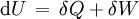In the case of an adiabatic change of state, there is no heat exchange with the environment (δQ = 0) so that the entire at the Work done in the system is completely converted into the internal energy or a part of the internal energy is completely converted into work from System is provided: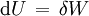This can greatly simplify calculations and theoretical considerations or, under certain circumstances, make them possible in the first place.

Both irreversible and reversible adiabatic changes of state are possible. In the first case, entropy is generated in the system during the process, in the latter case not. Because no heat energy is exchanged with the environment, no entropy flows in or out. If the change of state is reversible, the entropy of the system therefore remains the same; it is then an isentropic change of state. (The reverse does not apply: an isentropic change of state can also be irreversible and diabatic if exactly the generated entropy flows outwards; another counterexample is the behavior when approaching absolute temperature zero: Here the changes of state are due to the 3rd law of thermodynamics ( almost) always isentropic, but only in exceptional cases adiabatic).

Reversible adiabatic changes of state also play an important role in the axiomatic justification of thermodynamics according to Carathéodory. Starting from the axiom "In the vicinity of every reversibly achievable state there are states which are not adiabatically reversible, i.e. only irreversibly or not at all achievable" the existence of a new quantity of state entropy is proven. States between which only irreversible transitions are possible differ in their entropy, so that no adiabatic-reversible (i.e. isentropic) transition can exist between them.

### Approximate realization

An ideal adiabatic change of state presupposes that the system in which the change of state takes place is perfectly insulated against heat flows of any form. So heat conduction, convective heat transfer and radiation exchange would have to be completely prevented. A heat flow may flow through the system as long as no heat from it remains in the system; the heat flow can then be regarded as not belonging to the system (examples: a completely transparent system illuminated by the sun, a system flooded with non-interacting neutrinos).

In reality, complete thermal insulation cannot be achieved, but real processes can take place adiabatically to a good approximation if

• they take place in a well-insulated container (e.g. chemical reactions in an adiabatic calorimeter),
• if the change of state proceeds so quickly that little heat can flow in or out in the short time (e.g. in an internal combustion engine or when a spacecraft re-enters) or
• if the volume of the system is very large, so that heat flows at its edge play practically no role (e.g. with thermally rising air parcels).

In reality, it is practically always at least partially diabatic processes, so that one can only approximately assume an adiabatic change of state.

### Examples

• The compression of the air in a bicycle pump is an adiabatic change of state. If the compression is carried out at a sufficiently high speed, a significant increase in temperature can be felt. The work done on the pump directly increases the internal energy and thus the temperature of the air mixture. At first, no thermal energy is transferred to or absorbed by the pump. Only after the process has been completed do you notice a warming up of the bicycle pump and thus a flow of thermal energy. A pneumatic lighter uses this method. The extremely rapid primary heating of the air when spacecraft reenters is also an approximately adiabatic process due to the extremely high compression speeds. However, the heat is then distributed very quickly through conduction, flow and radiation processes.
• Conversely to compression, an adiabatic pressure drop causes the air to cool. This happens, for example, within an ascending air flow (with thermal lift or when flowing over a mountain range) or also on the upper side of aircraft wings. When cooling down, the saturation concentration for water vapor decreases. If this falls below the actual water content, the water above it condenses into small water droplets (fog formation).

### Working with a reversible adiabatic (isentropic) change of state of an ideal gas

In the case of an ideal gas, the following applies to the internal energy: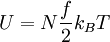Because of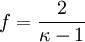follows: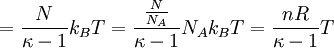.

Designate here N the number of gas particles, f the number of unfrozen degrees of freedom, kB. the Boltzmann constant, T the absolute temperature, κ the isentropic exponent, n the amount of substance (in moles), NA. the Avogadro constant and R. the general gas constant.

Hence, because of δW. = dU for the work performed during a reversible adiabatic (isentropic) change of state (volume work):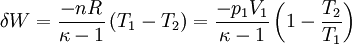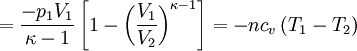.

Designate here T1,2 and V1,2 Initial and final temperatures and volumes, and cv the molar specific heat at constant volume.

It also follows that the work of the process with higher temperature difference T1T2 gets bigger. Among other things, this results in the adiabatic temperature gradient of the lower earth atmosphere.

These relationships follow from the equation of state of an ideal gas: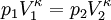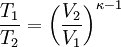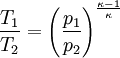These equations can be transformed in such a way that: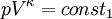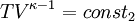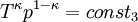They are also called Poisson's equations.

Since the mass of the gas volume also remains constant, the conversion to the change in density can also be easily calculated: